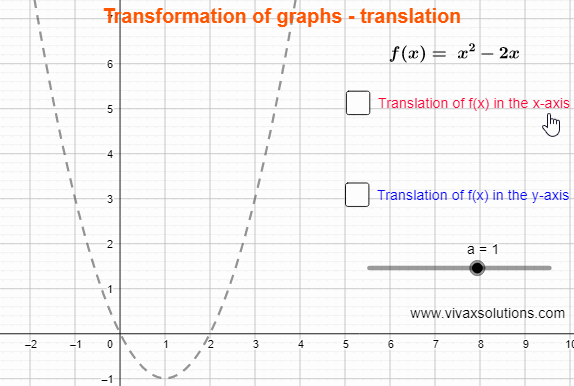### Transformation of Graphs - translation1. f(x) → f(x + a) - a translation in the negative x-axis by a
2. f(x) → f(x - a) - a translation in the positive x-axis by a
3. f(x) → f(x) +  a - a translation in the positive y-axis by a
4. f(x) → f(x) -  a - a translation in the negative y-axis by a

E.g.1

Sketch y = x2 + 2x - 3 and find the following:
1. The line of symmetry
2. The solutions when y = 0
3. The coordinates of the minimum point
4. The y-intercept

y = x2 + 2x - 3
By completing the square, y = (x + 1)2 - 4
1) Line of symmetry, x = -1
2) (x + 1)2 - 4 = 0 → x = -3 or x = 1
3) Turning point ~ (-1,-4)
4) y-intercept ~ (0,-3)

E.g.2

Sketch y = x2 -4x + 3 and find the following:
1. The line of symmetry
2. The solutions when y = 0
3. The coordinates of the minimum point
4. The y-intercept

y = x2 -4x + 3
By completing the square, y = (x - 2)2 - 1
1) Line of symmetry, x = 1
2) (x - 2)2 - 1 = 0 → x = 3 or x = 1
3) Turning point ~ (2,-1)
4) y-intercept ~ (0,3)

You can practise translation interactively with the following applet: